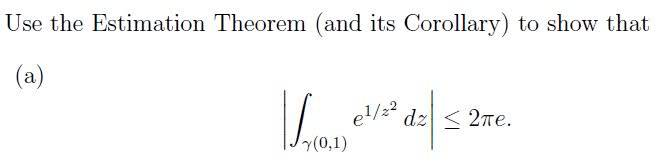# Contour Integral homeworkSo the length of the contour is L(gamma) = 2.pi

and so i have http://images.planetmath.org:8080/cache/objects/7138/js/img1.png

so i need to show max f(z) = e?

So the maximum of f(z)= e1/z2 in the unit circle centre 0, radius 1 implies that 1/z2 should be maximum, and this is when z2 is its lowest possible value.

When this happens f(z) cannot equal e unless the lowest possible value of z is 1, but isn't that the highest possible value?

Any help would be great thanks

#### Attachments

Last edited by a moderator:

On the unit circle z = exp(i theta). So you need to find an upper limit for

|exp[exp(-2 i theta)]|

Hint: Split the inner exponential in its imaginary and real parts.

On the unit circle z = exp(i theta). So you need to find an upper limit for

|exp[exp(-2 i theta)]|

Hint: Split the inner exponential in its imaginary and real parts.

so exp[cos(2t) - i.sin(2t)]

i have an example similar to this stage where i.sin(t) suddenly disappears, so I assume it does that here as well. Could you explain why for me please?

And then the upper bound of cos(2t) = 1 and so the result follows.

exp[cos(2t) - i.sin(2t)] = exp[cos(2t)] exp[- i.sin(2t)]

And then when you take the absolute value, you use that

|exp(i p)| = 1 for real p.

exp[cos(2t) - i.sin(2t)] = exp[cos(2t)] exp[- i.sin(2t)]

And then when you take the absolute value, you use that

|exp(i p)| = 1 for real p.

ah i didn't know that, thanks